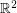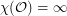# odd distance

## Coloring the Odd Distance Graph ★★★

Author(s): Rosenfeld

The Odd Distance Graph, denoted, is the graph with vertex setand two points adjacent if the distance between them is an odd integer.

Question   Is?

Keywords: coloring; geometric graph; odd distance